# Engineering Mechanics - Force System Resultants

### Exercise :: Force System Resultants - General Questions

• Force System Resultants - General Questions
31.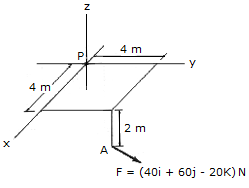Determine the moment of the force at A about point P. Use a vector analysis and express the result in Cartesian vector form.

 A. MP = (160i+240j+40k) N-m B. MP = (380i+160j+400k) N-m C. MP = (280i+200j+400k) N-m D. MP = (40i+80k) N-m

Explanation:

No answer description available for this question. Let us discuss.

32.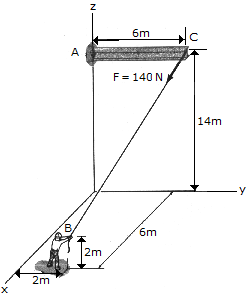The man at B exerts a force of 140N on the rope attached to the end of beam AC as shown. Determine the moment of this force about the base of the beam at A.

 A. MA = (720i-360k) N-m B. MA = (-720i+360k) N-m C. MA = (-720i-360k) N-m D. MA = (720i+360k) N-m

Explanation:

No answer description available for this question. Let us discuss.

33.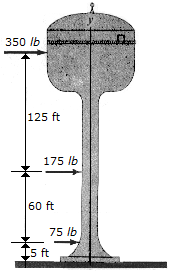The three forces acting on the water tank represent the effect of the wind. Replace this system by a single resultant force and specify its vertical location from point O.

 A. R = 600 lb, d = 65.0 ft below O B. R = 600 lb, d = 130.4 ft below O C. R = 600 lb, d = 65.0 ft above O D. R = 600 lb, d = 130.4 ft above O

Explanation:

No answer description available for this question. Let us discuss.

34.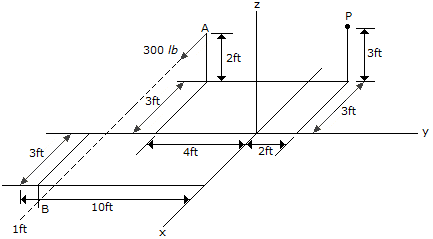Determine the moment of the force at A about point P. Use a vector analysis and express the result in Cartesian vector form.

 A. MP = (600i-200k) N-m B. MP = (800i-200j+1200k) N-m C. MP = (1200i+100k) N-m D. MP = (400i-200j+1200k) N-m

Explanation:

No answer description available for this question. Let us discuss.

35.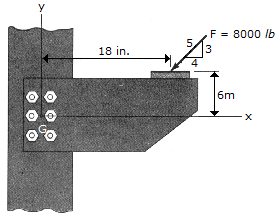This structural connection is subjected to the 8,000-lb force. Replace this force by an equivalent force and couple acting at the center of the bolt group, O.

 A. F = (6400i+4800j) lb, M = (-3.20i+7.20j) kip-ft B. F = (-6400i-4800j) lb, M = -400k kip-ft C. F = (-6400i-4800j) lb, M = (-3.20i-7.20j) kip-ft D. F = (6400i+4800j) lb, M = 400k kip-ft